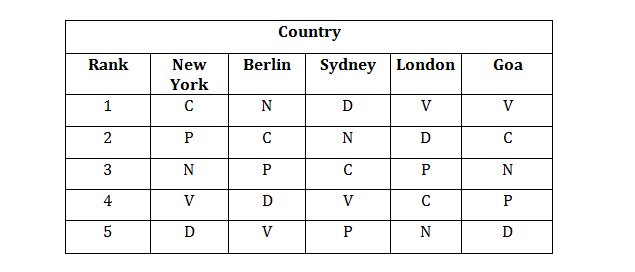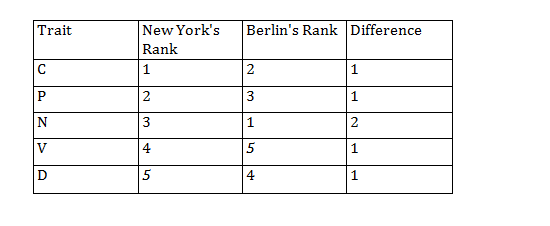Directions (Qs.1-4): Answer the questions on the basis of the information given below.
A study was conducted to ascertain the relative importance that employees in five different countries assigned to five different traits in their Chief Executive Officers. The traits were compassion (C), decisiveness (D), negotiation skills (N), public visibility (P), and Vision (V). The level of dissimilarity between two countries is the maximum difference in the ranks allotted by the two countries to any of the five traits. The following table indicates the rank order of the five traits for each country.Question 1: Which amongst the following countries is most dissimilar to New York?
(a) Berlin
(b) Sydney
(c) London
(d) Goa

Question 2: Which of the following pairs of countries are most dissimilar?
(a) Berlin & Sydney
(b) New York & Berlin
(c) London & Sydney
(d) Goa & Sydney

Question 3: Three of the following four pairs of countries have identical levels of dissimilarity. Which pair is the odd one out ?
(a) London & Berline
(b) Berline & Goa
(c)  Goa & Sydeney
(d) Sydeney & London

Question 4: Which of the following countries is least dissimilar to New york ?
(a) Berline
(b) Sydeney
(c) London
(d) Goa

Answers: Questions (1-4): Level of Dissimilarity = Maximum difference in ranks allotted to any two countries on any of the five traits. For example, dissimilarity between New York and BerlinFrom the above table the maximum difference is 2. ∴ The dissimilarity level between New York and Berlin is 2.

From above calculations, the highest level of dissimilarity is 4 which is for Sydney.

The level of dissimilarity between

Berlin and Sydney is for the parameter D (4 –1) = 3

New York and Berlin is for the parameter N (3 –1) = 2

London and Sydney is for the parameter V (4 – 1) = 3

Goa and Sydney is for the parameter D (5 –1) = 4

The level of dissimilarity between

London and Berline is for the parameter V (5 – 1) = 4

Berline and Goa is for the parameter V (5 –1) = 4

Goa and Sydeney is for the parameter D (5 –1) = 4

Sydeney and London is for the parameter V (4 – 1) = 3

Calculating the level of dissimilarity for New york Vs remaining countries

Berline : For the parameter N (3 –1) = 2

Sydeney : For the parameter D (5 –1) = 4

London : For the parameter C (4 –1) = 3

Goa : For the parameter V (4 –1) = 3

∴ The least level of dissimilarity is 2 which is for Berline.